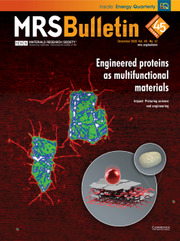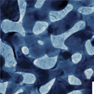Home
Hostname: page-component-65dc7cd545-fz4lj Total loading time: 0.243 Render date: 2021-07-25T17:27:04.917Z Has data issue: true Feature Flags: { "shouldUseShareProductTool": true, "shouldUseHypothesis": true, "isUnsiloEnabled": true, "metricsAbstractViews": false, "figures": true, "newCiteModal": false, "newCitedByModal": true, "newEcommerce": true, "newUsageEvents": true }MRS Bulletin

# Nanoporous metal by dealloying for electrochemical energy conversion and storage

Published online by Cambridge University Press:  10 January 2018

Corresponding

## AbstractMetallic materials are key for electrochemical energy conversion and storage when they are tailored into electrodes designed for rapid reaction kinetics, high electrical conductivities, and high stability. Nanoporous metals formed by dealloying could meet all of these requirements, as the dealloyed products beckon energy researchers with their fascinating structures and outstanding performance. In this article, we discuss the characteristics of dealloyed materials related to their functions in energy devices. We then review nanoporous metal electrodes for applications in fuel cells, supercapacitors, and batteries to provide insights into selection and design criteria for meeting the diverse needs of energy conversion and storage.

## Keywords

Type
Dealloyed Nanoporous Materials with Interface-Controlled Behavior
Information
MRS Bulletin , January 2018 , pp. 43 - 48

## Access options

Get access to the full version of this content by using one of the access options below.

## References

Ding, Y., Chen, M.W., Erlebacher, J., J. Am. Chem. Soc. 126, 6876 (2004).CrossRefGoogle Scholar
Lang, X.Y., Hirata, A., Fujita, T., Chen, M.W., Nat. Nanotechnol. 6, 232 (2011).CrossRefGoogle Scholar
Yu, Y., Gu, L., Lang, X., Zhu, C., Fujita, T., Chen, M., Maier, J., Adv. Mater. 23, 2443 (2011).CrossRefGoogle Scholar
Brandon, N., Brett, D., Philos. Trans. A. Math. Phys. Eng. Sci. 364, 147 (2006).CrossRefGoogle Scholar
Verbrugge, M.W., Liu, P., J. Electrochem. Soc. 152, D79 (2005).CrossRefGoogle Scholar
Conway, B.E., Electrochemical Supercapacitors: Scientific Fundamentals and Technological Applications, (Springer Science & Business Media, 2013).Google Scholar
Rahn, C.D., Wang, C.-Y., Battery Systems Engineering (Wiley, 2013).CrossRefGoogle Scholar
Artymowicz, D.M., Erlebacher, J., Newman, R.C., Philos. Mag. 89, 1663 (2009).CrossRefGoogle Scholar
Qian, L.H., Chen, M.W., Appl. Phys. Lett. 91, 83105 (2007).CrossRefGoogle Scholar
Qi, Z., Weissmüller, J., ACS Nano 7, 5948 (2013).CrossRefGoogle Scholar
Fujita, T., Guan, P., McKenna, K., Lang, X., Hirata, A., Zhang, L., Tokunaga, T., Arai, S., Yamamoto, Y., Tanaka, N., Ishikawa, Y., Asao, N., Yamamoto, Y., Erlebacher, J., Chen, M., Nat. Mater. 11, 1 (2012).CrossRefGoogle Scholar
Krekeler, T., Straßer, A.V., Graf, M., Wang, K., Hartig, C., Ritter, M., Weissmüller, J., Mater. Res. Lett. 5, 314 (2017).CrossRefGoogle Scholar
Mistry, H., Varela, A.S., Kühl, S., Strasser, P., Cuenya, B.R., Nat. Rev. Mater. 1, 16009 (2016).CrossRefGoogle Scholar
Wang, C., Markovic, N.M., Stamenkovic, V.R., ACS Catal. 2, 891 (2012).CrossRefGoogle Scholar
Wang, R., Liu, J.G., Liu, P., Bi, X.X., Yan, X.L., Wang, W.X., Ge, X.B., Chen, M.W., Ding, Y., Chem. Sci. 5, 403 (2014).CrossRefGoogle Scholar
Gasteiger, H.A., Kocha, S.S., Sompalli, B., Wagner, F.T., Appl. Catal. B 56, 9 (2005).CrossRefGoogle Scholar
Stamenkovic, V.R., Mun, B.S., Arenz, M., Mayrhofer, K.J.J., Lucas, C., Wang, G., Ross, P.N., Markovic, N.M., Nat. Mater. 6, 241 (2007).CrossRefGoogle Scholar
Koh, S., Strasser, P., J. Am. Chem. Soc. 129, 12624 (2007).CrossRefGoogle Scholar
Snyder, J., Fujita, T., Chen, M.W., Erlebacher, J., Nat. Mater. 9, 904 (2010).CrossRefGoogle Scholar
Xu, C., Wang, R., Chen, M.W., Zhang, Y., Ding, Y., Phys. Chem. Chem. Phys. 12, 239 (2010).CrossRefGoogle Scholar
Wang, R., Xu, C., Bi, X., Ding, Y., Energy Environ. Sci. 5, 5281 (2012).CrossRefGoogle Scholar
Wang, X., Frenzel, J., Wang, W., Ji, H., Qi, Z., Zhang, Z., Eggeler, G., J. Phys. Chem. C 115, 4456 (2011).CrossRefGoogle Scholar
Lang, X.Y., Han, G.F., Xiao, B.B., Gu, L., Yang, Z.Z., Wen, Z., Zhu, Y.F., Zhao, M., Li, J.C., Jiang, Q., Adv. Funct. Mater. 25, 230 (2015).CrossRefGoogle Scholar
Yu, J., Ding, Y., Xu, C., Inoue, A., Sakurai, T., Chen, M., Chem. Mater. 20, 4548 (2008).CrossRefGoogle Scholar
Chen, X., Si, C., Gao, Y., Frenzel, J., Sun, J., Eggeler, G., Zhang, G.Z., J. Power Sources 273, 324 (2015).CrossRefGoogle Scholar
Snyder, J., McCue, I., Livi, K., Erlebacher, J., J. Am. Chem. Soc. 134, 8633 (2012).CrossRefGoogle Scholar
Xu, C., Wang, L., Wang, R., Wang, K., Zhang, Y., Tian, F., Ding, Y., Adv. Mater. 21, 2165 (2009).CrossRefGoogle Scholar
Xu, C., Zhang, Y., Wang, L., Xu, L., Bian, X., Ma, H., Ding, Y., Chem. Mater. 21, 3110 (2009).CrossRefGoogle Scholar
Wang, R., Liu, J., Liu, P., Bi, X., Yan, X., Wang, W., Meng, Y., Ge, X., Chen, M., Ding, Y., Nano Res. 7, 1569 (2014).CrossRefGoogle Scholar
Yan, X., Meng, F., Xie, Y., Liu, J., Ding, Y., Sci. Rep. 2, 941 (2012).CrossRefGoogle Scholar
Ding, Y., Kim, Y.J., Erlebacher, J., Adv. Mater. 16, 1897 (2004).CrossRefGoogle Scholar
Wang, R., Wang, C., Cai, W., Ding, Y., Adv. Mater. 22, 1845 (2010).CrossRefGoogle Scholar
Neurock, M., Janikb, M., Wieckowskic, A., Faraday Discuss. 140, 363 (2008).CrossRefGoogle Scholar
Li, J., Yin, H.-M., Li, X.-B., Okunishi, E., Shen, Y.-L., He, J., Tang, Z.-K., Wang, W.-X., Yücelen, E., Li, C., Gong, Y., Gu, L., Miao, S., Liu, L.-M., Luo, J., Ding, Y., Nat. Energy 2, 17111 (2017).CrossRefGoogle Scholar
Guo, H., Yin, H., Yan, X., Shi, S., Yu, Q., Cao, Z., Li, J., Sci. Rep. 6, 39162 (2016).CrossRefGoogle Scholar
Lang, X.Y., Yuan, H.T., Iwasa, Y., Chen, M.W., Scr. Mater. 64, 923 (2011).CrossRefGoogle Scholar
Chen, L.Y., Kang, J.L., Hou, Y., Liu, P., Fujita, T., Hirata, A., Chen, M.W., J. Mater. Chem. A 1, 9202 (2013).CrossRefGoogle Scholar
Chen, L.Y., Hou, Y., Kang, J.L., Hirata, A., Fujita, T., Chen, M.W., Adv. Energy Mater. 3, 851 (2013).CrossRefGoogle Scholar
Hou, Y., Chen, L., Zhang, L., Kang, J., Fujita, T., Jiang, J., Chen, M., J. Power Sources 225, 304 (2013).CrossRefGoogle Scholar
Lang, X.Y., Zhang, L., Fujita, T., Ding, Y., Chen, M.W., J. Power Sources 197, 325 (2012).CrossRefGoogle Scholar
Kang, J., Hirata, A., Qiu, H.J., Chen, L., Ge, X., Fujita, T., Chen, M., Adv. Mater. 26, 269 (2014).CrossRefGoogle Scholar
Kang, J., Hirata, A., Kang, L., Zhang, X., Hou, Y., Chen, L., Li, C., Fujita, T., Akagi, K., Chen, M., Angew. Chem. Int. Ed. Engl. 52, 1664 (2013).CrossRefGoogle Scholar
Aricò, A.S., Bruce, P., Scrosati, B., Tarascon, J.-M., Van Schalkwijk, W., Nat. Mater. 4, 366 (2005).CrossRefGoogle Scholar
Rolison, D.R., Long, J.W., Lytle, J.C., Fischer, A.E., Rhodes, C.P., McEvoy, T.M., Bourg, M.E., Lubers, A.M., Chem. Soc. Rev. 38, 226 (2009).CrossRefGoogle Scholar
Guo, X., Han, J., Zhang, L., Liu, P., Hirata, A., Chen, L., Fujita, T., Chen, M.W., Nanoscale 7, 15111 (2015).CrossRefGoogle Scholar
Zhang, S., Xing, Y., Jiang, T., Du, Z., Li, F., He, L., Liu, W., J. Power Sources 196, 6915 (2011).CrossRefGoogle Scholar
Lu, Q., Rosen, J., Zhou, Y., Hutchings, G.S., Kimmel, Y.C., Chen, J.G., Jiao, F., Nat. Commun. 5, 3242 (2014).CrossRefGoogle Scholar
Peng, Z., Freunberger, S.A., Chen, Y., Bruce, P.G., Science 337 (6094), 563 (2012).CrossRefGoogle Scholar
Newman, J., Tiedemann, W., AIChE J. 21, 25 (1975).CrossRefGoogle Scholar

# Send article to Kindle

Note you can select to send to either the @free.kindle.com or @kindle.com variations. ‘@free.kindle.com’ emails are free but can only be sent to your device when it is connected to wi-fi. ‘@kindle.com’ emails can be delivered even when you are not connected to wi-fi, but note that service fees apply.

Find out more about the Kindle Personal Document Service.

Nanoporous metal by dealloying for electrochemical energy conversion and storage
Available formats
×

# Send article to Dropbox

To send this article to your Dropbox account, please select one or more formats and confirm that you agree to abide by our usage policies. If this is the first time you use this feature, you will be asked to authorise Cambridge Core to connect with your <service> account. Find out more about sending content to Dropbox.

Nanoporous metal by dealloying for electrochemical energy conversion and storage
Available formats
×

# Send article to Google Drive

To send this article to your Google Drive account, please select one or more formats and confirm that you agree to abide by our usage policies. If this is the first time you use this feature, you will be asked to authorise Cambridge Core to connect with your <service> account. Find out more about sending content to Google Drive.

Nanoporous metal by dealloying for electrochemical energy conversion and storage
Available formats
×
×